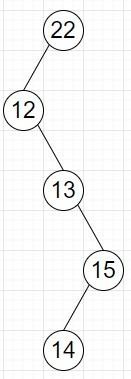# Check if each internal node of a BST has exactly one child in Python

Suppose we have the preorder traversal of a binary search tree (BST). We have to check whether each internal node has only one child or not.

So, if the input is like preorder = [22, 12, 13, 15, 14], then the output will be True as BST is like −To solve this, we can follow one efficient approach. As all decedents of a node is either smaller or larger, then we can we can follow these steps −

• Get the next preorder successor of the node

• Get the last preorder successor of the node

• Now when both the successors are less than or greater than the current node then check again otherwise return false.

To solve this, we will follow these steps −

• next := 0, last := 0
• for i in range 0 to size of preorder - 1, do
• next := preorder[i] - preorder[i+1]
• last := preorder[i] - last value of preorder
• if next * last < 0, then
• return False
• return True

Let us see the following implementation to get better understanding −

## Example

Live Demo

def solve(preorder):
next = 0
last = 0
for i in range(len(preorder)-1):
next = preorder[i] - preorder[i+1]
last = preorder[i] - preorder[-1]
if next * last < 0:
return False
return True
preorder = [22, 12, 13, 15, 14] print(solve(preorder))

## Input

[22, 12, 13, 15, 14]


## Output

True